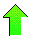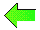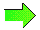ISSAC 94 - Symbolic-Numeric Nonlinear Equation SolvingContents News View

 ReferencesAlefeld, G., and Herzberger, J. (1983) Introduction to Interval Computations, Academic Press.

 Brent, R. P. (1971), "An Algorithm with Guaranteed Convergence for Finding a Zero of a Function", The Computer Journal, 14, 422-425.

 Bundy, A., and Welham, B. (1981) "Using Meta-Level Inference for Selective Application of Multiple Rewrite Rules in Algebraic Manipulation", Artificial Intelligence 16(2).

 Collins, G. E. and Loos, R. (1983) "Real Zeros of Polynomials", Computer Algebra Symbolic and Algebraic Computation, Second Edition, (ed. B. Buchberger, G. E. Collins, and R. Loos) Springer-Verlag, 83-94.

 Conway, John B. (1978), Functions of One Complex Variable, Second Edition, Springer-Verlag.

 Hardy, G. H. (1910), Orders of infinity.  Cambridge Tracts in Mathematics 12.

 Hoenders, B. J. and Slump, C. H. (1992) "On the Determination of the Number and Multiplicity of Zeros of a Function", Computing, 47(3-4), 323-336.

 Ioakimidis, N. I. and Anastasselou, E. G. (1986) "On the Simultaneous Determination of Zeros of Analytic or Sectionally Analytic Functions", Computing 36(3) 239-247.

 Kearfott, R. Baker (1991) "Decomposition of Arithmetic Expressions to Improve the Behaviour of Interval Arithmetic for Nonlinear Systems", Computing 47(2), 169-191.

 Nerinckx, D., and Haegemans, A. (1976), "A Comparison of Non-Linear Equation Solvers", Journal of Computational and Applied Mathematics 2, 145-148.

 Ratschek, H., and Rokne, J. (1984) Computer Methods for the Range of Functions, Ellis Horwood Limited.

 Richardson, Daniel (1968) "Some Undecidable Problems Involving Elementary Functions of a Real Variable", The Journal of Symbolic Logic 33(4), 514-520.

 Rice, John R. (1993) Numerical Methods, Software, and Analysis, Academic Press, 323-410.

 Rudin, Walter (1976), Principles of Mathematical Analysis, McGraw-Hill.

 Saaty, Thomas L. (1981), Modern Nonlinear Equations, Dover.